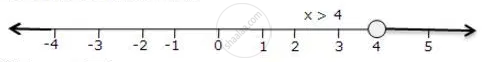# Represent the Solution of the Given Inequalities on the Real Number Line 4x - 1 > X + 11 - Mathematics

Represent the solution of the given inequalities on the real number line

4x - 1 > x + 11

#### Solution

4x - 1 > x + 11

3x > 12

x > 4

The solution on number line isConcept: Linear Inequations in One Variable
Is there an error in this question or solution?

#### APPEARS IN

Selina Concise Maths Class 10 ICSE
Chapter 4 Linear Inequations (In one variable)
Exercise 4 (B) | Q 4.1 | Page 49